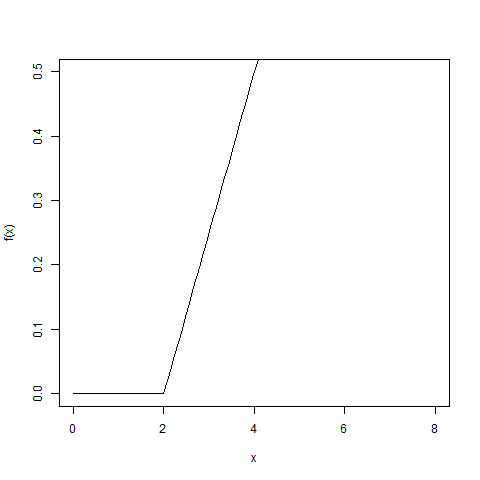# Compute the value of CDF on Uniform Distribution in R Programming – punif() Function

In R programming, `punif()` function is used to compute the value of Cumulative Distribution Function(CDF).

Syntax:
punif(q, min = 0, max = 1, lower.tail = TRUE, log.p = FALSE)

Parameters:
q: represents vector of quantiles
min, max: represents lower and upper limits of the distribution
lower.tail: represents logical value. If TRUE, probabilities are P[X<=x]
log.p: represents logical value. If TRUE, probabilities are given as log(p)

Example 1:

 `# Create vector of random deviation  ` `u <- ``runif``(20) ` ` `  `punif``(u) == u ` ` `  `print``(``punif``(u)) `

Output:

```  TRUE TRUE TRUE TRUE TRUE TRUE TRUE TRUE TRUE TRUE TRUE TRUE TRUE TRUE TRUE
 TRUE TRUE TRUE TRUE TRUE

 0.21571509 0.97224483 0.74654757 0.93053902 0.90868119 0.93048128
 0.41566382 0.52074950 0.41353715 0.48460207 0.63706965 0.16338451
 0.22761876 0.54239105 0.07045675 0.04363406 0.68484316 0.86928257
 0.06046589 0.29565811
```

Example 2:

 `# Output to be present as PNG file  ` `png``(file = ``"punifGFG.png"``)  ` `   `  `# Plot function  ` `curve``(``punif``(x, min = 2, max = 6), 0, 8, ylim = ``c``(0, 0.5),  ` `      ``ylab = ``"f(x)"``)  ` `   `  `# Saving the file  ` `dev.off``()  `

Output:My Personal Notes arrow_drop_upCheck out this Author's contributed articles.

If you like GeeksforGeeks and would like to contribute, you can also write an article using contribute.geeksforgeeks.org or mail your article to contribute@geeksforgeeks.org. See your article appearing on the GeeksforGeeks main page and help other Geeks.

Please Improve this article if you find anything incorrect by clicking on the "Improve Article" button below.

Article Tags :

Be the First to upvote.

Please write to us at contribute@geeksforgeeks.org to report any issue with the above content.